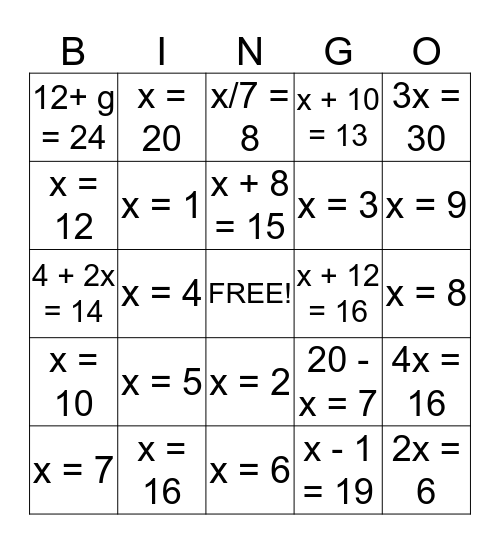# Algebra BingoThis bingo card has a free space and 24 words: x = 5, x = 16, x + 8 = 15, x = 7, 3x = 30, 12+ g = 24, x = 12, x = 20, x + 12 = 16, x = 2, x = 3, 4 + 2x = 14, 20 - x = 7, 4x = 16, x = 1, x - 1 = 19, x = 8, x/7 = 8, x = 9, x = 4, 2x = 6, x = 10, x + 10 = 13 and x = 6.

## Play Online